• ## 日期排序

千次阅读 2020-02-26 09:45:01
有一些日期日期格式为“MM/DD/YYYY”。编程将其按日期大小排列。 Input Output Sample Input 12/12/1999 10/21/2003 10/22/2003 02/12/2004 11/30/2005 12/31/2005 Sample Output 12/12/1999 10/21/2003 10/22/...
在这里插入代码片
Description
有一些日期，日期格式为“MM/DD/YYYY”。编程将其按日期大小排列。
Input
Output
Sample Input
12/12/1999
10/21/2003
10/22/2003
02/12/2004
11/30/2005
12/31/2005
Sample Output
12/12/1999
10/21/2003
10/22/2003
02/12/2004
11/30/2005
12/31/2005
#include<stdio.h> #include<stdlib.h> struct riqi{  int month,day,year; }data; int comp(const void *p1,const void *p2) {  struct riqi *c = (struct riqi *)p1;  struct riqi *d = (struct riqi *)p2;  if(c->year != d->year)  {   return d->year < c->year?1:-1;  }  else if(c->month != d->month&&c->year == d->year)  {   return d->month < c->month?1:-1;  }  else  {   return d->day < c->day?1:-1;  } } int main(void) {  int i = 0;  while(scanf("%d/%d/%d",&data[i].month,&data[i].day,&data[i].year) != EOF)  {   i++;  }  qsort(data,i,sizeof(data),comp);  for(int n = 0;n < i;n++)  {   printf("%02d/%02d/%02d\n",data[n].month,data[n].day,data[n].year);  } }
展开全文• 要求用户任意输入几个日期，再输入当天日期，筛选出小于当天日期日期，再把这些日期以从先到后的顺序排列。 求助各路大神![图片说明](http://forum.csdn.net/PointForum/ui/scripts/csdn/Plugin/001/face/21.gif)...
• ## C语言 通讯录排序

万次阅读 2017-06-29 13:52:15
任务描述 ...随后n行，每行按照“姓名 生日 电话号码”的格式给出一位朋友的信息，其中“姓名”是长度不超过10的英文字母组成的字符串，“生日”是yyyymmdd格式的日期，“电话号码”是不超过17位的数字
任务描述  输入n个朋友的信息，包括姓名、生日、电话号码，本题要求编写程序，按照年龄从大到小的顺序依次输出通讯录。题目保证所有人的生日均不相同。  功能要求  ①输入格式:  输入第一行给出正整数n（<<10）。随后n行，每行按照“姓名 生日 电话号码”的格式给出一位朋友的信息，其中“姓名”是长度不超过10的英文字母组成的字符串，“生日”是yyyymmdd格式的日期，“电话号码”是不超过17位的数字及+、-组成的字符串。  ②输出格式:  按照年龄从大到小输出朋友的信息，格式同输出。  ③输入样例:  3  zhang 19850403 13912345678  wang 19821020 +86-0571-88018448  qian 19840619 13609876543  ④输出样例:
wang 19821020 +86-0571-88018448  qian 19840619 13609876543  zhang 19850403 13912345678
#include<stdio.h>
#include<stdlib.h>
typedef struct {
char name;
long int birth;
char phone;
}pInfo;

int pInfoSort(pInfo *data, int n)
{
int i, j;
pInfo temp;
for (i = 0; i < n; i++) {
for (j = i + 1; j < n; j++) {
if (data[i].birth > data[j].birth) {
temp = data[i];
data[i] = data[j];
data[j] = temp;
}
}
}
for (i = 0; i < n; i++) {
printf("%s  ", data[i].name);
printf("%ld  ", data[i].birth);
printf("%s\n", data[i].phone);
}
return 0;
}

int main()
{
pInfo *data;
int n, i;
scanf("%d", &n);
data = (pInfo*)malloc(sizeof(pInfo) * n);
for (i = 0; i < n; i++) {
scanf("%s", data[i].name);
scanf("%ld", &data[i].birth);
scanf("%s", data[i].phone);
}
pInfoSort(data, n);
system("pause");
return 0;
}
展开全文• 问题来源于成绩统计，名次排序 1.定义一个结构体 typedef struct Stu{ char name; int id; int score; }stu; 2.定义排序（回调）函数： /*定义排序函数*/ int cmp(const void *a,const void ...
问题来源于成绩统计，名次排序

1.定义一个结构体

typedef struct Stu{
char name;
int id;
int score;
}stu;


2.定义排序（回调）函数：

/*定义排序函数*/
int cmp(const void *a,const void *b){
stu c = *(stu*)a;
stu d = *(stu*)b;
//printf("%d\n",strcmp(c.name,d.name));
if(strcmp(c.name,d.name)>0){/*返回值是0、1*/<br>      return strcmp(c.name,d.name);
}
else{
if(strcmp(c.name,d.name)==0){
return c.id-d.id;
}
}
}

或更精简
int cmp(const void *c,const void *d){
return *(int *)c - *(int *)d;
}


3.使用qsort函数 qsort(st,n,sizeof(st),cmp); 头文件：stdlib.h 用 法： void qsort(void *base,int nelem,int width,int (*fcmp)(const void *,const void *)); 参数： 1 :待排序数组首地址; 2 :数组中待排序元素数量; 3 :单个元素的大小,推荐使用sizeof(st)这样的表达式; 4 :指向函数的指针，用于确定排序的顺序.

一般采用思路
#include<stdio.h>
#include<string.h>
int main()
{
int n;
char name;
char sex;
char year;
int score;

int max = -1;
int mix = 200;
/*最高分者信息*/
char maxname;
char maxsex;
char maxyear;
/*最低分者信息*/
char mixname;
char mixsex;
char mixyear;

scanf("%d",&n);
for(int i=0;i<n;i++){
scanf("%s",name);
scanf("%s",sex);
scanf("%s",year);
scanf("%d",&score[i]);
/*若当前输入的分数比mix小，则将此条信息记录为最低分者*/
if(score[i]<mix){
strcpy(mixname,name);
strcpy(mixsex,sex);
strcpy(mixyear,year);
mix = score[i];
}
/*若当前输入的分数比max大，则将此条信息记录为最高分者*/
if(score[i]>max){
strcpy(maxname,name);
strcpy(maxsex,sex);
strcpy(maxyear,year);
max = score[i];
}
}
printf("\n最高者：%s\t性别：%s\t年龄：%s\n",maxname,maxsex,maxyear);
printf("最低者：%s\t性别：%s\t年龄：%s\n",mixname,mixsex,mixyear);
}


#include<stdio.h>
#include<string.h>
#include<stdlib.h>
#include<math.h>
#include<ctype.h>
/*定义一个结构体*/
typedef struct Stu{
char name;
char sex;
int age;
int score;
}stu;
/*    定义排序(回调)函数cmp：
返回类型必须是int;
两个参数的类型必须都是const void *;
如果是升序,那么就是如果a比b大返回一个正值,小则负值,相等返回0;
*/
int cmp(const void *a,const void *b){
/* *(stu*)a是因为：a是个void *类型，要先
用(stu*）将它转成stu*类型，然后再用*取值，
变成stu类型，才能比较大小。*/
stu c=*(stu*)a;
stu d=*(stu*)b;
//按成绩升序排序
return c.score-d.score;
}
main(){
int n;
stu sz;
scanf("%d",&n);
for(int i=0;i<n;i++){
scanf("%s %s %d %d",&sz[i].name,&sz[i].sex,&sz[i].age,&sz[i].score);
}
/*
qsort函数参数：
1 待排序数组首地址;
2 数组中待排序元素数量;
3 各元素的占用空间大小,推荐使用sizeof(s)这样,特别是对结构体 ;
4 指向函数的指针，用于确定排序的顺序.
注意：如果要对数组进行部分排序,比如对一个s[n]的数组排列其从s[i]开始的m个元素,只需要
在第一个和第二个参数上进行一些修改:qsort(&s[i],m,sizeof(s[i]),cmp);
*/
qsort(sz,n,sizeof(sz),cmp);
printf("\n按成绩升序为：\n\n");
for(int i=0;i<n;i++){
printf("%s %s %d %d\n",sz[i].name,sz[i].sex,sz[i].age,sz[i].score);
}
}

[转自] (https://www.cnblogs.com/panweiwei/p/6481034.html)
展开全文• 十大排序算法基本思想，动画演示及其C语言实现，不要再找了，我看了好多博客里面都是有问题的。本文代码手打，已经过测试，不涉及算法复杂度以及优化，仅供理解概念。
十大排序算法基本思想及其C语言实现

写在前面：欢迎阅读本文，博主小王系国内大数据专业本科大二菜鸟一名，开始写博客出于兴趣以及试图记录自己的学习情况，主要写作范围是数据结构与算法,大数据,机器学习,c/c++ (部分内容尚未开始),更多博客请访问博客主页，如有兴趣可关注，一起学习。

前几天想看一下排序算法，结果好几篇博客里都有问题，于是自己重新写了一篇，水平有限，此处不涉及算法复杂度及其优化，只是为了更好的理解。 下面的99%的代码都是手动敲出来的，参考了诸多资料，已经经过测试，可以放心食用。
推荐学习网站:
菜鸟教程-十大经典排序算法 (有的地方还是讲的稍微晦涩,但是提供了多种语言实现,代码可能对初学者略难理解)
动画来源:菜鸟教程-十大经典排序算法

文章目录
十大排序算法基本思想及其C语言实现1.冒泡排序2.选择排序3.插入排序4.希尔排序5.归并排序6.快速排序7.堆排序8.计数排序9.桶排序10.基数排序

1.冒泡排序
基本思想
冒泡排序基本思想是依次比较两个相邻的元素，如果顺序（如从大到小、首字母从Z到A）错误就把他们交换过来。走访元素的工作是重复地进行直到没有相邻元素需要交换，也就是说该元素列已经排序完成。
在进行第一轮上面的从左到右的比较时，则会把一个最小或者最大的元素（取决于你想要的排列方式）"冒泡"到最右边的位置，第二轮则是冒泡第二大或第二小的数到最右边，因此我们总共只需要进行n-1轮即可，最后一个数的位置也被固定了（其余n-1个数都比他大且都在其右边）。
这个算法的名字由来是因为越小的元素会经由交换慢慢“浮”到数列的顶端（升序或降序排列），就如同碳酸饮料中二氧化碳的气泡最终会上浮到顶端一样，故名“冒泡排序”。 动画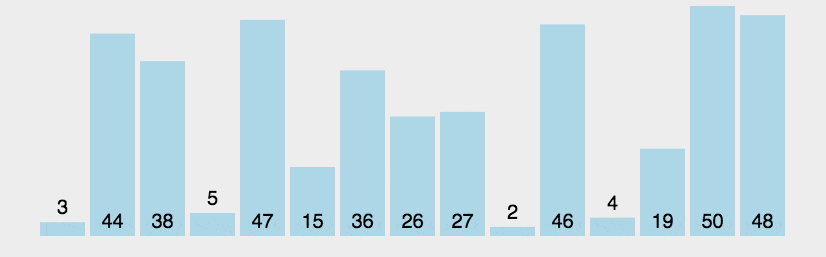实现
void bubbleSort()
{
//C实现
int arr[] = {5, 9, 3, 8, 6};
int len = sizeof(arr)/sizeof(arr);
int temp;
for (int i = 0; i < len - 1; i++) //从小到大
{                                 // 外循环为排序趟数，len个数进行len-1趟
for (int j = 0; j < len - 1 - i; j++)
{ // 内循环为每趟比较的次数，第i趟比较len-i次,因为第一次已经将最大的元素冒泡到最后一个位置了
if (arr[j] > arr[j + 1])
{ //相邻元素比较,逆序则将交换位置
temp = arr[j];
arr[j] = arr[j + 1];
arr[j + 1] = temp;
}
}
}

//打印数组
for (int i = 0; i < len; i++)
printf("%d\t", arr[i]);
}


2.选择排序
基本思想
第一次从待排序的数据元素中选出最小（或最大）的一个元素，存放在序列的起始位置，然后再从剩余的未排序元素中寻找到最小（大）元素，然后放到已排序的序列的末尾。以此类推，直到全部待排序的数据元素的个数为零。 动画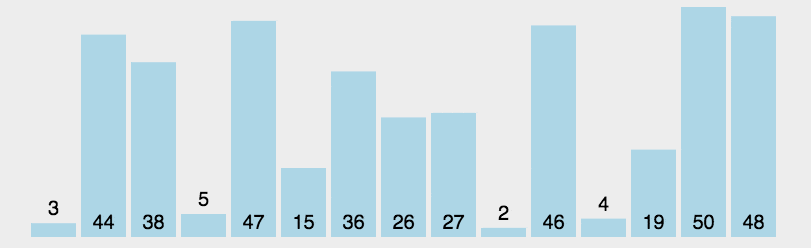实现
  int arr[] = {100, 92, 5, 9, 3, 8, 23, 17, 50, 6};
int len = sizeof(arr)/sizeof(arr);
int index = 0;                //待会用来存储未排序区最小元素的位置索引
for (int i = 0; i < len; i++) //从小到大
{
index = i;
for (int j = i + 1; j < len; j++) //用i之后的每一个元素去与i元素比较大小,若小于arr[i]则更新最小元素索引
{
if (arr[j] < arr[index])
index = j;
}
//将i与index的元素调换位置
//注意:此处不可将调换位置的函数写进第二层for循环即for(int j=i+1)中,因为交换后i与min指向的对象会交换,此后循环就可能出现仅仅小于arr[i](此时已经换到了min位置)但不小于arr[min](这时在i位置上)的元素也与初始位置上进行交换的情况,具体情况可以试验!
if (i != index) //判断是否需要调换,将最小元素位置换至第一个未排序的位置
{
int temp = arr[i];
arr[i] = arr[index];
arr[index] = temp;
}
}

//打印数组
for (int i = 0; i < len; i++)
printf("%d\t", arr[i]);
}

3.插入排序
基本思想
插入排序是一种最简单的排序方法，它的基本思想是将一个记录插入到已经排好序的有序表中，从而形成一个新的、记录数增1的有序表。
一般将第一个元素看做最小的有序组,然后用第二个元素插入到第一个元素组成的有序表中(其实就是个简单的比较大小),然后将第三个元素插入到前两个元素组成的有序表中,形成一个三个元素的有序表,以此类推,最终获得一个包含全部元素的有序表实现
void insertionSort()
{
int arr[] = {1, 5, 3, 9, 7};
int len = sizeof(arr)/sizeof(arr);

int i, j, key;
for (i = 1; i < len; i++) //从1开始是因为默认第一个元素是最小的有序表
{
key = arr[i];
j = i - 1; //a[j]是有序表最后一个元素
while ((j >= 0) && (arr[j] > key)) //从后往前遍历并且判断arr[j]是否大于key
//j>=0防止数组越界
{
arr[j + 1] = arr[j];//后移
j--;//j前移
}
arr[j + 1] = key; //arr[j]是第一个比key小的元素,将key置于其后(比key大的有序表元素都已经后移一个位置了)
}

//打印数组
for (int i = 0; i < len; i++)
printf("%d\t", arr[i]);
}

4.希尔排序
基本思想
希尔排序，也称递减增量排序算法，是插入排序的一种更高效的改进版本
基本思想是先将整个待排序的记录序列分割成为若干子序列分别进行直接插入排序，待整个序列中的记录"基本有序"时，再对全体记录进行依次直接插入排序。
具体实现方法:
把待排序列，分成多个间隔为gap的子序列，  然后对每个子序列进行插入排序  重复上述，每次间隔gap不同（并且越来越小），直到最后一次选取间隔gap=1，完成排序。  我们一般是取数组长度的一半为第一个间隔，即第一次将整个数组以len/2为间隔分为2个子序列，再进行插入排序，然后以len/2/2为间隔一直到gap=1重复上述动作 下图来自网络,便于理解实现
void shellSort()
{

int arr[] = {1,12,13,19,26,7,8,15,3,23,99,8,35,27,34,5};
int len = sizeof(arr)/sizeof(arr);  //16

int gap, i, j;
int temp;

for (gap = len / 2; gap >= 1; gap /= 2) //第一个间隔为len/2,然后不断除2缩小
{
for (i = gap; i < len; i++) //对每一个下标大于gap的元素进行遍历
//arr[gap]是第一组最后一个元素
{
temp = arr[i]; //将要插入的值赋值给temp,因为它所处的位置可能被覆盖
for (j = i - gap; arr[j] > temp && j >= 0; j -= gap)
{                         //i所处的子序列:i  i-gap  i-2gap i-n*gap( i-n*gap  >= 0)
arr[j + gap] = arr[j]; //arr[j]若大于要插入的值则将位置后移
}
arr[j + gap] = temp; //无论是arr[j]<temp还是j<0了,都将temp插入到arr[j]这一个子序列的后一个位置(j+gap)
}
}

//打印数组
for (int i = 0; i < len; i++)
printf("%d\t", arr[i]);
}

B站参考视频:希尔排序-哔哩哔哩
5.归并排序
基本思想
说实话我今天刚看的时候没太搞懂…
这篇博客写的蛮好的,可以参照-传送门.
动画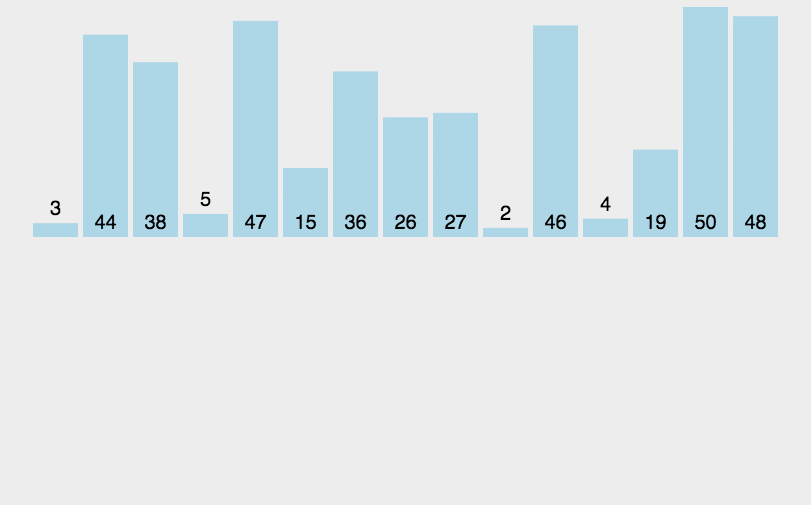实现
int min(int x, int y)
{
return x < y ? x : y;
}
void merge_sort(int arr[], int len)
{
int *a = arr;                              //左指针->首元素
int *b = (int *)malloc(len * sizeof(int)); //右指针->尾元素
int seg, start;
for (seg = 1; seg < len; seg += seg)
{
for (start = 0; start < len; start += seg * 2)
{
int low = start;
int mid = min(start + seg, len);
int high = min(start + seg * 2, len);

int k = low;
int start1 = low, end1 = mid;
int start2 = mid, end2 = high;
while (start1 < end1 && start2 < end2)
b[k++] = a[start1] < a[start2] ? a[start1++] : a[start2++];
while (start1 < end1)//将左边剩下的元素放置到数组b中
b[k++] = a[start1++];
while (start2 < end2)//将左边剩下的元素放置到数组b中
b[k++] = a[start2++];
}
int *temp = a;
a = b;
b = temp;
}
if (a != arr)
{
int i;
for (i = 0; i < len; i++)
b[i] = a[i];
b = a;
}
free(b);
}

6.快速排序
基本思想
从数列中挑出一个元素，称为 “基准”（pivot）;  重新排序数列，所有元素比基准值小的摆放在基准前面，所有元素比基准值大的摆在基准的后面（相同的数可以到任一边）。在这个分区退出之后，该基准就处于数列的中间位置。这个称为分区（partition）操作；  递归地（recursive）把小于基准值元素的子数列和大于基准值元素的子数列排序；
动画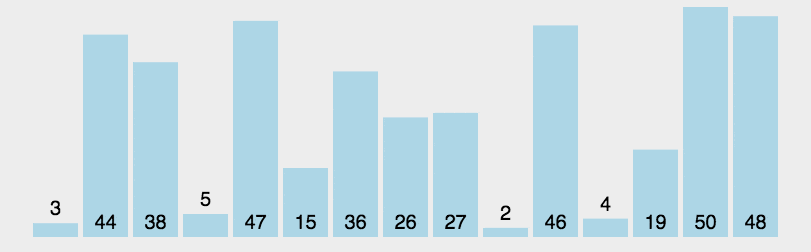实现
void quickSort()
{
int arr = {11, 7, 9, 3, 4, 6, 2, 8, 5, 3};
quick_sort(arr, 0, 9);
for (int i = 0; i < 10; i++)
printf("%d\t", arr[i]);
}

int partition(int arr[], int start, int end)
{
int temp = arr[start];//以arr[start]作为基准,将被放置在数组中间
//同时将start位置作为交换元素的缓冲点-类比交换两个数的第三个数
int li = start, ri = end;//li->left index 左索引 li==start 所以初始li是第一个缓冲点
while (li < ri)
{
while (li < ri && arr[ri] > temp)//找到我们右起第一个小于基准值的元素索引ri
ri--;
if (li < ri)
{
arr[li] = arr[ri];//将右起第一个小于基准值的元素索引放置在缓冲点(li)
//同时此时的ri成为新的缓冲点
li++;
}
while (li < ri && arr[li] < temp)//找到我们右起第一个小于基准值的元素索引li
li++;
if (li < ri)
{
arr[ri] = arr[li];//将左起第一个大于基准值的元素索引放置在缓冲点 (ri)
//同时此时的li成为新的缓冲点
ri--;
}
//结束上述操作后li和ri分别是左右已排序部分(置于两端)的后面一个和前面一个元素(不包含在其中)
//明显若li==ri则只剩下最后一个位置
}
arr[li] = temp;
return li;//返回的是基准值最终的索引
}

void quick_sort(int arr[], int start, int end)
{
if (start < end)
{
int index = partition(arr, start, end);//依照基准值分区
quick_sort(arr, start, index - 1);//基准值之左再排序
quick_sort(arr, index + 1, end);//基准值之右再排序
}
}

7.堆排序
基本思想
创建一个大顶堆( 每个结点的值都大于或等于其左右孩子结点的值，称为大顶堆 )；  把堆首（最大值）和堆尾互换；  把堆的尺寸缩小 1，利用剩下的元素再次建立一个大顶堆；  重复步骤 2 3，直到堆的尺寸为 1。 推荐博客:图解算法之堆排序 B 站视频:堆排序算法
动画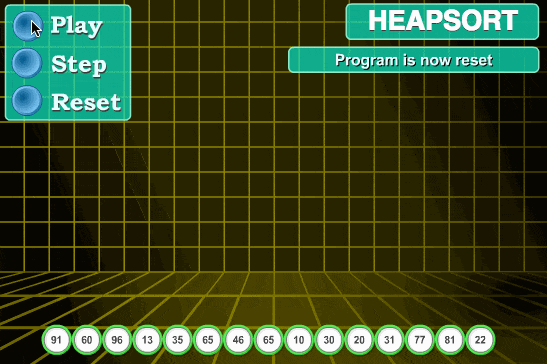实现
//7.堆排序
void heapSort()
{
int arr[] = {3, 5, 3, 0, 8, 6, 1, 5, 8, 6, 2, 4, 9, 4, 7, 0, 1, 8, 9, 7, 3, 1, 2, 5, 9, 7, 4, 0, 2, 6};
int len = (int)sizeof(arr) / sizeof(*arr);
for (int i = len; i > 1; i--)
heap_Sort(arr, i); //建立堆 每次规模减1

//打印结果
for (int i = 0; i < len; i++)
printf("%d ", arr[i]);
return 0;
}

//构造一个大顶堆并将最大值换至最后一位
void heap_Sort(int arr[], int len)
{
int dad = len / 2 - 1; //最后一个父节点
int son = 2 * dad + 1; //该父节点下的首个子节点
{
//判断是否有两个子节点若有则在其中寻找最大子节点
if (son + 1 <= len - 1 && arr[son] < arr[son + 1])
son++;
son = 2 * dad + 1; //上一个父节点的首个子节点
}
swap(&arr, &arr[len - 1]);
}

void swap(int *a, int *b)
{
int temp = *a;
*a = *b;
*b = temp;
}

8.计数排序
基本思想
找出待排序的数组中最大和最小的元素统计数组中每个值为i的元素出现的次数，存入数组C的第i项对所有的计数累加（从C中的第一个元素开始，每一项和前一项相加）反向填充目标数组：将每个元素i放在新数组的第C(i)项，每放一个元素就将C(i)减去1
动画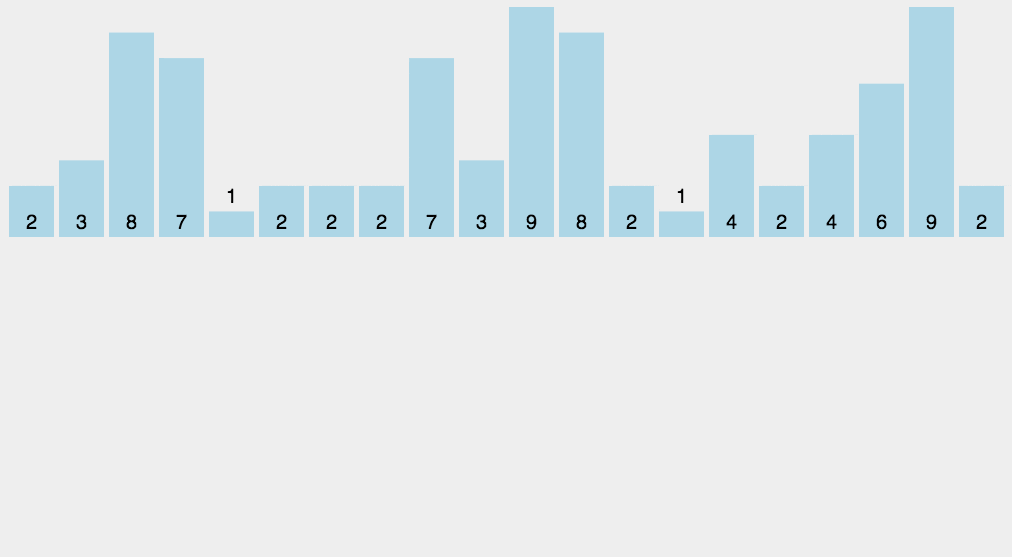实现
void countingSort()
{
int arr[] = {3, 5, 3, 0, 8, 6, 1, 5, 8, 6, 2, 4, 9, 4, 7, 0, 1, 8, 9, 7, 3, 1, 2, 5, 9, 7, 4, 0, 2, 6};
int len = (int)sizeof(arr) / sizeof(*arr);
counting_Sort(arr, len);

for (int i = 0; i < len; i++)
printf("%d   ", arr[i]);
}

void counting_Sort(int arr[], int LEN)
{
//寻找最大最小值
int max = arr, min = arr, i, j = 0;
for (i = 0; i < LEN; i++)
{
if (arr[i] < min)
min = arr[i];
if (arr[i] > max)
max = arr[i];
}

//建立计数数组
int new_len = max - min + 1;

int conunting_arr[new_len];

for (i = 0; i < new_len; i++) //初始化
conunting_arr[i] = 0;

for (i = 0; i < LEN; i++)	//计数
conunting_arr[arr[i] - min]++;

//根据计数结果进行排序
for (i = 0; i < new_len; i++)
{
int index = conunting_arr[i];
while (index != 0)
{
arr[j] = i + min;
index--;
j++;
}
}
}


9.桶排序
最简单的桶排序
将数组{1,3,3,7,9,2,4,6,6,0}进行排序:
观察数组元素范围，看出来是从0到9（可以去遍历取得最大最小值），所以我们建立10个有序桶，将数字一个个塞入对应的桶中，然后根据桶的情况进行输出（桶中有几个元素就输出几个，没有就跳过）-实际上就是最简单的计数排序，但网上有人把这个也算作桶排序了，不要搞混，下面来看真正的桶排序吧。
基本思想
桶排序(Bucket sort)或所谓的箱排序，计数排序的升级版，工作的原理是将数组分到有限数量的桶子里。 每个桶子再个别排序（有可能再使用别的排序算法或是以递归方式继续使用桶排序进行排序）。
每个桶对应一个数据或者一个数据段(实际上当每个桶对应一个数据的时候其实就是前面的计数排序)
这里我们使每个桶对应一个数据段并且对桶中元素使用冒泡排序进行排序操作
动画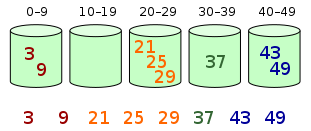实现
void bucketSort()
{
int arr[] = {3, 5, 3, 0, 8, 6, 1, 5, 8, 6, 2, 4, 9, 4, 7, 0, 1, 8, 9, 7, 3, 1, 2, 5, 9, 7, 4, 0, 2, 6};
int len = (int)sizeof(arr) / sizeof(arr);//30
bucket_Sort(arr, len);

for (int i = 0; i < len; i++)
printf("%d   ", arr[i]);
}

void bucket_sort(int arr[], int LEN)
{
int bucket = {0}, i, j, k, temp; //初始化桶,每个桶存放6个数据
//寻找最大最小值
int min = arr, max = arr;
for (i = 0; i < LEN; i++)
{
if (arr[i] < min)
min = arr[i];
if (arr[i] > max)
max = arr[i];
}
//遍历数组,将元素放到对应桶中
int index0 = 0, index1 = 0, index2 = 0, index3 = 0, index4 = 0;
for (i = 0; i < LEN; i++)
{
if (arr[i] < min + (max - min + 1) / 5 * 1 && index0 < 7)
{
bucket[index0] = arr[i];
index0++;
}
else if (arr[i] < min + (max - min + 1) / 5 * 2 && (index1 < 7 || index0 >= 7))
{
bucket[index1] = arr[i];
index1++;
}
else if (arr[i] < min + (max - min + 1) / 5 * 3 && (index2 < 7 || index1 >= 7))
{
bucket[index2] = arr[i];
index2++;
}
else if (arr[i] < min + (max - min + 1) / 5 * 4 && (index3 < 7 || index2 >= 7))
{
bucket[index3] = arr[i];
index3++;
}
else if (arr[i] < min + (max - min + 1) / 5 * 5 && (index4 < 7 || index3 >= 7))
{
bucket[index4] = arr[i];
index4++;
}
}

//在每个桶中使用冒泡排序
for (i = 0; i < 5; i++)
{
for (int j = 0; j < 5; j++) //从小到大
{                           // 外循环为排序趟数，len个数进行len-1趟
for (int k = 0; k < 5 - i; k++)
{ // 内循环为每趟比较的次数，第i趟比较len-i次,因为第一次已经将最大的元素冒泡到最后一个位置了
if (bucket[i][k] > bucket[i][k + 1])
{ //相邻元素比较,逆序则将交换位置
temp = bucket[i][k];
bucket[i][k] = bucket[i][k + 1];
bucket[i][k + 1] = temp;
}
}
}
}

//将桶中排序结果还原到原数组中
for (i = 0; i < 5; i++)
{
for (j = 0; j < 6; j++)
{
arr[i * 6 + j] = bucket[i][j];
}
}
}

10.基数排序
基本思想
基数排序是一种非比较型整数排序算法，其原理是将整数按位数切割成不同的数字，然后按每个位数分别比较。由于整数也可以表达字符串（比如名字或日期）和特定格式的浮点数，所以基数排序也不是只能使用于整数。
动画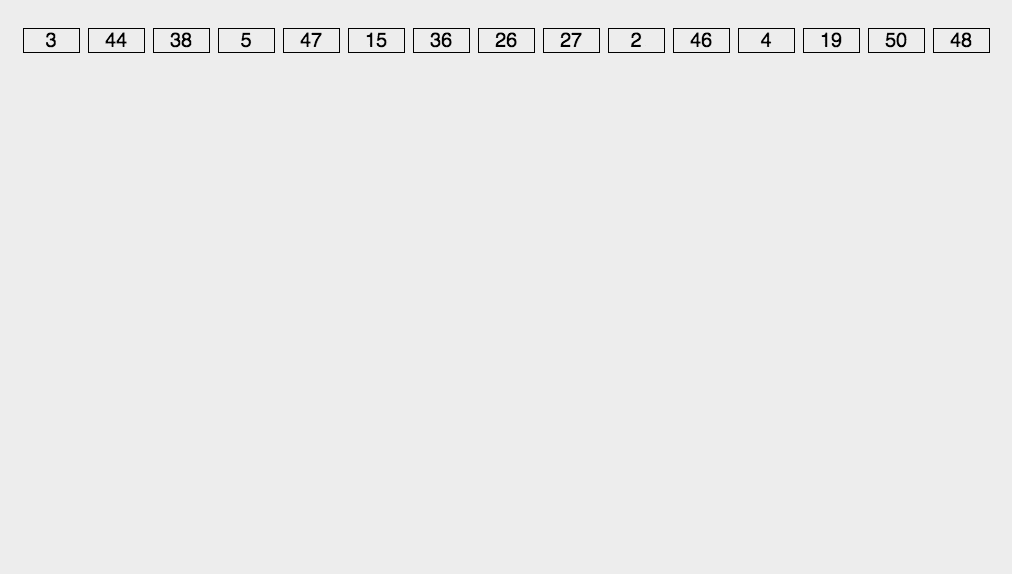实现
#include <stdio.h>

{
int b[n], m = a, exp = 1, BASE = 10, i;

//寻找最大值
for (i = 1; i < n; i++)
if (a[i] > m)
m = a[i];

while (m / exp > 0)
{
int bucket[BASE] = {0};
//按照exp所在的位将所有元素放入桶中
for (i = 0; i < n; i++)
bucket[(a[i] / exp) % BASE]++;
//做前缀和-以<=i结尾的数的个数-以i结尾的数的最大索引+1
for (i = 1; i < BASE; i++)
bucket[i] += bucket[i - 1];
//依据前缀和将原数组中每一个元素放置在基于技术排列后的位置 并将前缀和减1
for (i = n - 1; i >= 0; i--)
b[--bucket[(a[i] / exp) % BASE]] = a[i];
//复制回原数组
for (i = 0; i < n; i++)
a[i] = b[i];

exp *= BASE;
}
}

int main()
{
int arr = {1, 35, 98, 256, 789, 47, 4, 956, 64, 222};
int len = sizeof(arr) / sizeof(arr);

for (int i = 0; i < len; i++)
printf("%d\t", arr[i]);

return 0;
}


# 如果您觉得文章尚有帮助,欢迎点赞关注收藏三连,大家的支持都是对小王的鼓励,更多内容可前往博客主页
数据结构与算法 2.大数据 3. 机器学习 4. c/c++
# 注：如果文章有任何问题或 迷惘不清之处，欢迎拍砖指正提出。
展开全文算法
• /* ============================================================================= 相关知识介绍（所有定义只为帮助读者理解相关概念，并非严格定义）： 1、稳定排序和非稳定排序 简单地说就是所有相等语言 c 算法 insert 测试
• C语言：万能排序（函数指针的运用）(代码皆在linux下gcc编译) 我们在学习一门语言时，最为普遍的就应该属实现排序的功能了，但是一旦要比较的参数类型发生了变化，我们就得重写一个排序，这样子很麻烦，效率也不高，...指针
• 本文件中讲述了c语言经典的282个案例，由浅入深。有利于提高广大爱好c语言编程的人员。 其中包括： 第1章 初识C语言 1 实例001 第一个C语言程序 2 实例002 一个完整的C语言程序 2 实例003 输出名言 3 实例004 用TC ...
• 通讯录排序 题目 输入n个朋友的信息，包括姓名、生日、电话号码，本题要求编写程序，按照年龄从大到小的顺序依次输出通讯录。题目保证所有人的生日均不相同。 输入格式: 输入第一行给出正整数n（<10）。随后n行，...算法
• printf("请输入待排序的数列: \n"); for(i =0;i;i++) { scanf("%d ",(Array+i)); } printf("待排序的数列是： \n"); for(i=0;i;i++) { printf("%d ",*(Array+i)); } QuickSort(Array,0,n-1); printf(...
• 　安全局搜索到了一批(n个)身份证号码，希望按出生日期对它们进行从大到小排序，如果有相同日期，则按身份证号码从小到大进行排序。身份证号码为18位的数字组成，出生日期为第7到第14位 　 解题思路： 整个思路很...字符串
• 1.3　因为C语言没有精确定义类型的大小，所以我一般都用typedef定义int16和int32。然后根据实际的机器环境把它们定义为int、short、long等类型。这样看来，所有的问题都解决了，是吗？ 1.4　新的64位机上的64位类型...
• 这是利用c语言中的链表来解决的问题，有利于你对C语言链表的更好的了解
• 随机生成10000个随机数，进行冒泡排序和快速排序，并比较时间。 #include<stdlib.h> #include<stdio.h> #include<time.h> void BubbleSort(int *a, int length) //冒泡 { for (int i = 0; i &...
• 　安全局搜索到了一批(n个)身份证号码，希望按出生日期对它们进行从大到小排序，如果有相同日期，则按身份证号码大小进行排序。身份证号码为18位的数字组成，出生日期为第7到第14位 输入格式 　第一行一个整数n，...
• 排序算法常见的有八种： 算法一：插入排序 插入排序是一种最简单直观的排序算法，它的工作原理是通过构建有序序列，对于未排序数据，在已排序序列中从后向前扫描，找到相应位置并插入。 算法步骤： 1）将第一待...数据结构
• 系统能够完成员工信息的查询、更新、插入、删除、排序等功能。 基本要求：排序：按不同关键字，对所有员工的信息进行排序；查询：按特定条件查找员工；更新，按编号对某个员工的某项信息进行修改；插入，加入新员工...
• 随后n行，每行按照“姓名 生日 电话号码”的格式给出一位朋友的信息，其中“姓名”是长度不超过10的英文字母组成的字符串，“生日”是yyyymmdd格式的日期，“电话号码”是不超过17位的数字及+、-组成的字符串。...
• *文件名称：排序.c *作者： 一只普通的圆 *完成日期：2021年2月20日 *编译工具：devc++ *版本号： v5.11 * *问题描述：输入10个数字，求和并输出 *程序输出：10个由大到小排列的数 */ #include<stdio.h> ...
• C语言冒泡排序 1.问题简介 　冒泡排序是一种将一组数据按照大小规律重新排列的算法，其简单易上手但计算量往往较大。 ２.算法分析 　相邻数据依次比较，将较大数防止后方，最后将经过多次循环将数字从大到小排列，注...算法
• ”，这次HJS大牛准备调查一下，于是收集了那里每个人的年龄数据，首先得把它们从小到大排序，这个简单的任务就交给你了！ 输入 第一行一个n，表示n个数(n&lt;=6000000) 第二行有n个数，表示该地区每个人的年龄...
• 有时候需要遍历目录文件，但是默认的函数并不支持指定排序的功能，现在介绍一种排序的遍历目录方式，默认按照文件名的数字进行排序 window版本 string split_pathexe(string szFullPath)//获取文件名 {  char ...
• 输入日期判断第几天 5.输入整数进行排序 6.用*号显示字母C的图案 7.显示特殊图案 8.打印九九口诀 9.输出国际象棋棋盘 10.打印楼梯并按条件打印笑脸 11.经典兔子问题 12.判断素数 13.水仙花数问题 14.正整数分解质...
• 直接插入排序示例: 此处以数据2的排序为例，用i从左到右遍历到下标为5的位置，发现此处的值2小于前一位的值5 下标 0 1 2 3 4 5 6 数据 1 3 4 5 2 6 遍历位置 i 将2放到缓存0的位置，然后数据5...数据结构
• 小明希望将自己的通讯录按好友的生日排序，这样就查看起来方便多了，也避免错过好友的生日。为了小明的美好愿望，你帮帮他吧。小明的好友信息包含姓名、出生日期。其中出生日期又包含年、月、日三部分信息。输入n个...
• 折半插入排序示例: 此处以数据2的排序为例，用i从左到右遍历到下标为5的位置，发现此处的值2小于前一位的值5 下标 0 1 2 3 4 5 6 数据 1 3 4 5 2 6 遍历位置 i 将2放到缓存0的位置，下限low从...数据结构
• ## PTA：通讯录排序（C语言）

千次阅读 多人点赞 2020-04-19 14:59:05
随后n行，每行按照“姓名 生日 电话号码”的格式给出一位朋友的信息，其中“姓名”是长度不超过10的英文字母组成的字符串，“生日”是yyyymmdd格式的日期，“电话号码”是不超过17位的数字及+、-组成的字符串。...
• 小明希望将自己的通讯录按好友的生日排序排序，这样就查看起来方便多了，也避免错过好友的生日。为了小明的美好愿望，你帮帮他吧。小明的好友信息包含姓名、出生日期。其中出生日期又包含年、月、日三部分信息。输入...
• C语言课程设计-球队管理系统 一，前言 这个是我学期末的一项C语言课设作业，经过大佬点播，身为小白的我终于完成了它，鉴于博客中还没有关于此方面（球队信果息管理）的内容，先把我的作业在此分享给大家。仅作为......

# c语言日期排序c语言 订阅